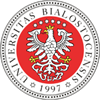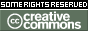REPOZYTORIUM UNIWERSYTETU
W BIAŁYMSTOKUProszę używać tego identyfikatora do cytowań lub wstaw link do tej pozycji: `http://hdl.handle.net/11320/5499`
 Tytuł: Tarski Geometry Axioms – Part II Autorzy: Coghetto, RolandGrabowski, Adam Słowa kluczowe: Tarski’s geometry axiomsfoundations of geometryEuclidean plane Data wydania: 2016 Data dodania: 16-maj-2017 Wydawca: De Gruyter Open Źródło: Formalized Mathematics, Volume 24, Issue 2, pp. 157–166 Abstrakt: In our earlier article , the first part of axioms of geometry proposed by Alfred Tarski  was formally introduced by means of Mizar proof assistant . We defined a structure TarskiPlane with the following predicates: of betweenness between (a ternary relation),of congruence of segments equiv (quarternary relation), which satisfy the following properties: congruence symmetry (A1),congruence equivalence relation (A2),congruence identity (A3),segment construction (A4),SAS (A5),betweenness identity (A6),Pasch (A7). Also a simple model, which satisfies these axioms, was previously constructed, and described in . In this paper, we deal with four remaining axioms, namely: the lower dimension axiom (A8),the upper dimension axiom (A9),the Euclid axiom (A10),the continuity axiom (A11). They were introduced in the form of Mizar attributes. Additionally, the relation of congruence of triangles cong is introduced via congruence of sides (SSS).In order to show that the structure which satisfies all eleven Tarski’s axioms really exists, we provided a proof of the registration of a cluster that the Euclidean plane, or rather a natural  extension of ordinary metric structure Euclid 2 satisfies all these attributes.Although the tradition of the mechanization of Tarski’s geometry in Mizar is not as long as in Coq , first approaches to this topic were done in Mizar in 1990  (even if this article started formal Hilbert axiomatization of geometry, and parallel development was rather unlikely at that time ). Connection with another proof assistant should be mentioned – we had some doubts about the proof of the Euclid’s axiom and inspection of the proof taken from Archive of Formal Proofs of Isabelle  clarified things a bit. Our development allows for the future faithful mechanization of  and opens the possibility of automatically generated Prover9 proofs which was useful in the case of lattice theory . Afiliacja: Coghetto Roland - Rue de la Brasserie 5, 7100 La Louvière, BelgiumGrabowski Adam - Institute of Informatics, University of Białystok, Ciołkowskiego 1M, 15-245 Białystok, Poland URI: http://hdl.handle.net/11320/5499 DOI: 10.1515/forma-2016-0008 ISSN: 1426-26301898-9934 Typ Dokumentu: Article Występuje w kolekcji(ach): Artykuły naukowe (WMiI)Formalized Mathematics, 2016, Volume 24, Issue 2

Pliki w tej pozycji:
Plik Opis RozmiarFormat
Pozycja ta dostępna jest na podstawie licencji Licencja Creative Commons CCL